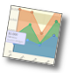Active Graphs and ChartsDynamic Data from Databases

In this tutorial we show how to use the graphing package to plot data directly from database in real time.

The bar graph below is plotting data from a MySQL databases which contains sales data. Upon the load of this page the graph will issue a request (via an Ajax call) to a server script for the data. The server script will read the data from the database and return to the graph software. The values are then plotted on the graph.

Configure the Graph

The first step is to configure the graph software to request the data from a server script. This is done with the parameter 'JpGraphDataFile'. Here is the configuration used for this graph:-

Create the Data Script

For this example we have created the data script with PHP. The server side script can be written in any server side language and at the bottom of this page there are sample scripts for ASP, JSP and PHP

The script is fairly straight forward and performs the following steps:-

• Connect to the Database
• Issue the Query
• Read through the results and output in the format required for the graph

Formatting the Data Results

In the above example we are reading monthly data for 3 products from the database. For each product we have a series of data, in this case 12 values representing each month of the year. The data should be output in the following format:-

where 'M' is the series number and N the data number. Here is a sample output of the script:-

Sample Data Scripts

The server script may be written in any language, here are sample scripts for some common languages:-

Database Schema

Here is the database schema used in this example:-

back to tutorial index